Next: Estimation in the presence Up: .  Introduction: Variable gain image Previous: Previous work on variable

## Quantimetric imaging

The quantity, denoted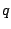, of light, to which an image sensor responds, is known as the photoquantity  which is neither radiometric nor photometric, but nevertheless provides quantifiable units of light. Quantimetric imaging, also known as (photo)quantigraphic imaging  measures the quantity of light in units that depend on the spectral response of the particular sensor used in a particular camera, rather than in units of flat spectral response (radiometry) or in units of the human eye's response (photometry).

Differently exposed images (e.g. individual frames of video) of the same subject matter are denoted as vectors: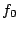,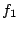,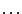,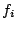,,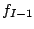,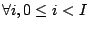.

Each video frame is some unknown function,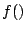, of the actual quantity of light,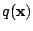falling on the image sensor: f_i = f(k_i q ( A_ix+b_i c_ix+d_i )), where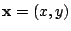denotes the spatial coordinates of the image,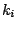is a single unknown scalar exposure constant, and parameters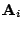,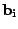,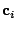, and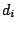denote the projective coordinate transformation between successive pairs of images: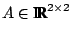is the linear coordinate transformation (e.g. accounts for magnification in each of the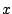and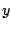directions and shear in each of theanddirections),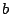is the translation in each of these two coordinate directions, and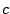is the projective chirp rate in each of these two coordinate directions. The additional constant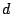makes the coordinate transformation into a group.

For simplicity, this coordinate transformation is assumed to be able to be independently recovered (e.g. using the methods of ). Therefore, without loss of generality, in this paper, it will be taken to be the identity coordinate transformation, which corresponds to the special case of images differing only in exposure.

Without loss of generality,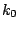will be called the reference exposure, and will be set to unity, and frame zero will be called the reference frame, so that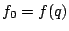. Thus we have: 1k_i f^-1 (f_i) = f^-1 (f_0), i, 0<i<I.

Taking the logarithm of both sides, F^-1 (f_i) - K_i = F^-1 (f_0), i, 0<i<I, where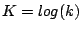, and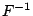is the logarithmic inverse camera response function (e.g. a LookUp Table converting pixel values into exposure values).

Re-arranging, we have: F^-1 (f_i) - F^-1 (f_0) = K_i, i, 0<i<I. This relation suggests a way to estimate the camera response function,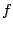, from a pair of differently exposed images of the same subject matter. Before estimating the camera response function, we consider how the noise will affect the estimation.Next: Estimation in the presence Up: .  Introduction: Variable gain image Previous: Previous work on variable
Steve Mann 2002-05-25